Why neural networks don’t work and how to use them

# Neural networks as universal model approximators

We can think of a neural network, $$\mathbb{NN}(\boldsymbol{w}, \boldsymbol{\alpha}) : {\bf d}\to\boldsymbol{\tau}$$, as an approximation of a model, $$\mathcal{M} : {\bf d}\to{\bf t}$$, where $${\bf d}$$ is some input data to the network and the output of the network is $$\boldsymbol{\tau}$$ which is an estimate of some target, $${\bf t}$$, associated with the data. The neural network itself is a function of some trainable parameters called weights, $$\boldsymbol{w}$$, and some hyperparameters, $$\boldsymbol{\alpha}$$, which encompass the architecture of the network, the initial values of the weights, the form of activation functions, the choice of cost function, etc.

# Likelihood of obtaining targets given a network

In a traditional sense, the training of a neural network is equivalent to minimising a cost or loss function, $$\Lambda({\bf t}, \boldsymbol{\tau})$$, with respect to the weights of the network, $$\boldsymbol{w}$$ (and hyperparameters, $$\boldsymbol{\alpha}$$) given a set of pairs of data and targets for training and validation, $$\{ {\bf d}^\textrm{train}_i, {\bf t}^\textrm{train}_i\vert i\in[1, n_\textrm{train}]\}$$ and $$\{ {\bf d}^\textrm{val}_i, {\bf t}^\textrm{val}_i\vert i\in[1, n_\textrm{val}]\}$$. The cost function, $$\Lambda({\bf t}, \boldsymbol{\tau})$$, measures how close the outputs of a fixed network, $$\mathbb{NN}(\boldsymbol{w}^*,\boldsymbol{\alpha}^*) : {\bf d}\to\boldsymbol{\tau}$$, are to some target, $${\bf t}$$, given a data-target pair, $$\{ {\bf d}, {\bf t}\}$$, at some fixed network parameters and hyperparameters, $$\boldsymbol{w}=\boldsymbol{w}^*$$ and $$\boldsymbol{\alpha}=\boldsymbol{\alpha}^*$$. That is, how likely is it that the output of the network provides the true target for the input data given a chosen set of weights and fixed network hyperparameters, i.e. the cost function is equivalent to the (negative logarithm of the) likelihood function

$\Lambda({\bf t}, \boldsymbol{t})\simeq-\textrm{ln}\mathcal{L}({\bf t}\vert {\bf d},\boldsymbol{w}^*,\boldsymbol{\alpha}^*).$The likelihood surface, although regular for a given set of network parameters and hyperparameters, is extremely complex, degenerate, and even discrete and non-convex in the directions of the network parameters and hyperparameters.

Although the cost function is normally chosen to be convex, i.e. with a global minimum and defined everywhere, at a given value of $$\boldsymbol{w}=\boldsymbol{w}^*$$ and $$\boldsymbol{\alpha}=\boldsymbol{\alpha}^*$$, the shape of the likelihood is extremely complex, degenerate and bumpy when considering all possible $$\boldsymbol{w}$$ and will often be discrete and non-convex in the $$\boldsymbol{\alpha}$$ direction.

## Maximum likelihood network parameter estimates

The normal procedure for using neural networks is to train them. This means finding the maximum likelihood estimates of the weights of a network with a given set of training data-target pairs $$\{ {\bf d}^\textrm{train}_i, {\bf t}^\textrm{train}_i\vert i\in[1, n_\textrm{train}]\}$$ and fixed hyperparameters, $$\boldsymbol{\alpha}=\boldsymbol{\alpha}^*$$, by doing

$\boldsymbol{w}^\textrm{MLE}=\underset{\boldsymbol{w}}{\textrm{argmax} }\left[\mathcal{L}(\{ {\bf t}^\textrm{train}_i\vert i\in[1, n_\textrm{train}]\}\vert \{ {\bf d}^\textrm{train}_i\vert i\in[1, n_\textrm{train}]\}, \boldsymbol{w}, \boldsymbol{\alpha}^*)\right].$

That is, find the set of $$\boldsymbol{w}$$ for which the likelihood function evaluated at every member in the training set is maximum. In the case that each pair of data and targets, $$\{ {\bf d}, {\bf t}\}$$ are independent and identically distributed we can write the likelihood as

$\mathcal{L}(\{ {\bf t}^\textrm{train}_i\vert i\in[1, n_\textrm{train}]\}\vert \{ {\bf d}^\textrm{train}_i\vert i\in[1, n_\textrm{train}]\}, \boldsymbol{w}, \boldsymbol{\alpha}^*)=\prod_i^{n_\textrm{train} }\mathcal{L}({\bf t}^\textrm{train}_i\vert {\bf d}^\textrm{train}_i, \boldsymbol{w},\boldsymbol{\alpha}).$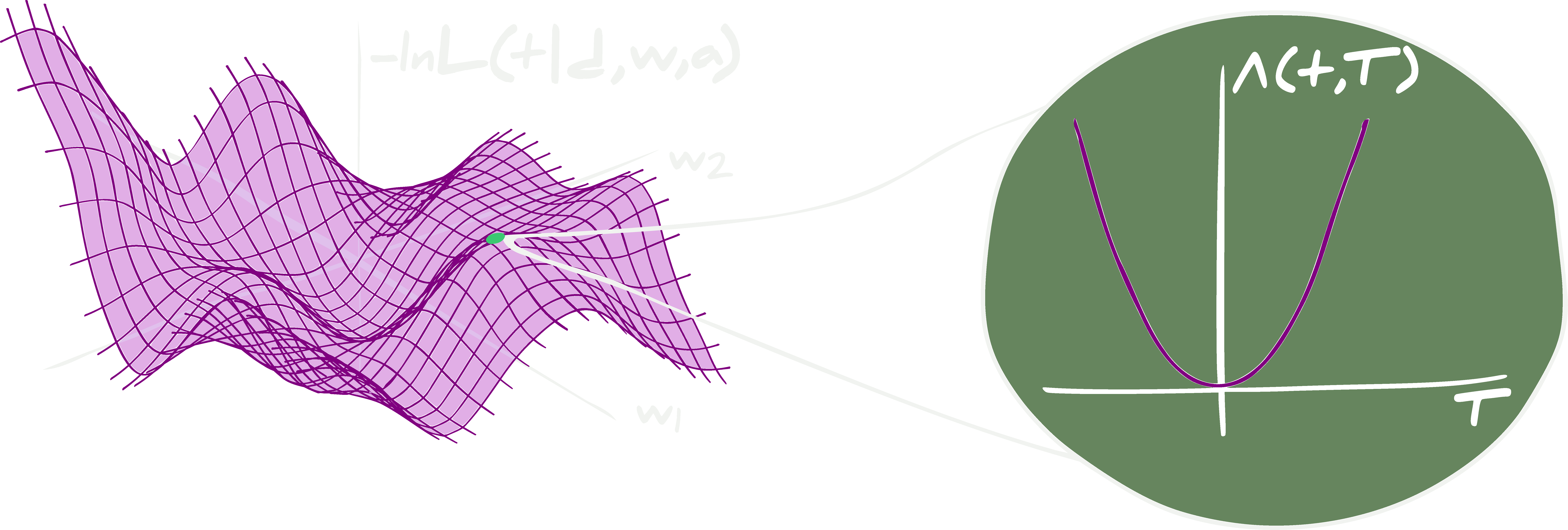By finding the set of $$\boldsymbol{\tau}$$ which are closest (in the sense of the minimum cost function) to the target $${\bf t}$$, given some a neural network and some input data $${\bf d}$$, the weights of the network traverse the negative logarithm of the likelhiood surface for the true target, hopefully ending at some minimum (which is a maximum in the likelihood).

To find the maximum likelihood of the weights, one would normally consider some sort of stochastic gradient descent. Since most software is more efficient at finding minima rather than maxima, we actually minimise the negative logarithm of the likelihood, i.e. the cost function

\begin{align} \boldsymbol{w}^\textrm{MLE}&=\underset{\boldsymbol{w}}{\textrm{argmin} }\left[\sum_i^{n_\textrm{train} }\Lambda({\bf t}^\textrm{train}_i, \boldsymbol{\tau}^\textrm{train}_i)\right]\\ &=\underset{\boldsymbol{w}}{\textrm{argmin} }\left[-\sum_i^{n_\textrm{train} }\textrm{ln}\mathcal{L}({\bf t}^\textrm{train}_i\vert {\bf d}^\textrm{train}_i, \boldsymbol{w}, \boldsymbol{\alpha}^*)\right]. \end{align}

The weights are updated using $$\boldsymbol{w}\to\boldsymbol{w}-\nabla_\boldsymbol{w} \sum_i^{n_\textrm{train} }\ \textrm{ln}\mathcal{L}({\bf t}^\textrm{train}_i\vert {\bf d}^\textrm{train}_i, \boldsymbol{w}, \boldsymbol{\alpha}^*)$$. In the ideal case there would be one global minimum in the likelihood so that after training the value of the weights of the neural network would be equal to the maximum likelihood estimates, $$\boldsymbol{w}=\boldsymbol{w}^\textrm{MLE}$$. However, since the likelihood surface is, in reality, extremely degenerate and flat in the space of weight values, it is most likely that the weights only achieve a local maximum, i.e. $$\boldsymbol{w}=\boldsymbol{w}^\textrm{local MLE}$$. In fact, which local maximum is found will normally depend extremely strongly on the initial $$\boldsymbol{w}=\boldsymbol{w}_\textrm{init}$$ which is used for the gradient descent.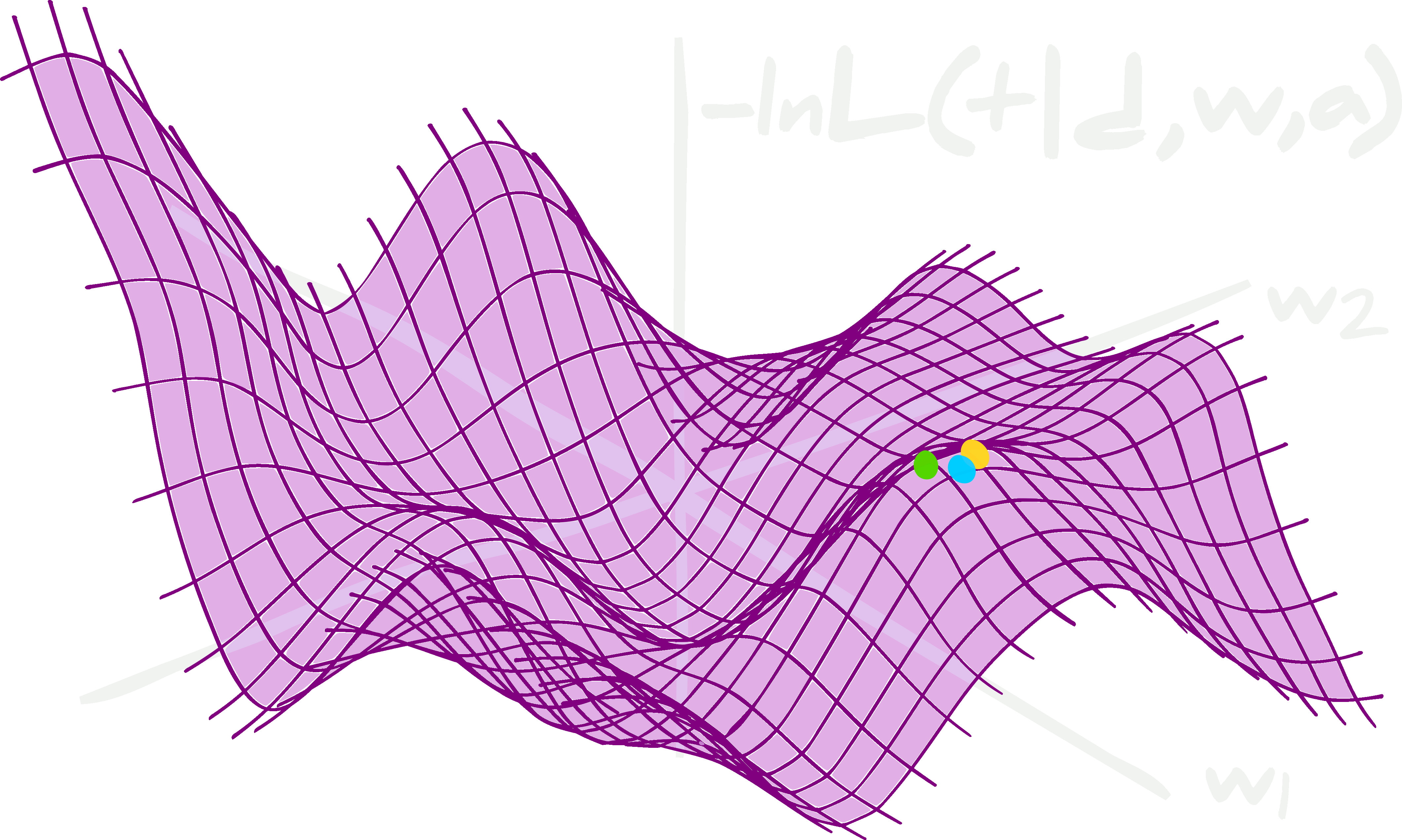The initialisation of the weights will be very important in determining which local maximum likelihood estimate is found. This is because the surface of the likelihood is very bumpy. It can also be highly degenerate which leads to whole families of pseudo-maximum likelihood estimates.

Once the maximum (or at least local maximum) is found, it is normal to evaluate the accuracy (or some other figure of merit) using some validation set, $$\{ {\bf d}^\textrm{val}_i, {\bf t}^\textrm{val}_i\vert i\in[1, n_\textrm{val}]\}$$. This validation set is used to modify the hyperparameters, $$\boldsymbol{\alpha}$$, of the network to achieve the best fit to both the training and validation sets as possible. These modifications could include changing the initial seeds of the weights, changing the activation functions, or changing the entire architecture, for example. However, networks trained in such a way do not provide a way to obtain scientifically robust estimates of the true targets $${\bf t}$$, given observed data $${\bf d}$$. To see why, we need to consider the probabilistic interpretation of neural networks.

# Probabilistic interpretation of neural networks

The posterior predictive density of obtaining a target, $${\bf t}$$, given some input data, $${\bf d}$$, is

$\mathcal{P}({\bf t}\vert {\bf d}) = \int d\boldsymbol{w}d\boldsymbol{\alpha}~\mathcal{L}({\bf t}\vert {\bf d}, \boldsymbol{w}, \boldsymbol{\alpha})\mathcal{P}(\boldsymbol{w},\boldsymbol{\alpha}).$

The likelihood of obtaining the true value of the target $$\mathcal{L}({\bf t}\vert {\bf d}, \boldsymbol{w},\boldsymbol{\alpha})$$, which is the (unnormalised) negative exponential of the cost function, when given some input data $${\bf d}$$ and network parameters and hyperparameters $$\boldsymbol{w}$$ and $$\boldsymbol{\alpha}$$. $$\mathcal{P}(\boldsymbol{w}, \boldsymbol{\alpha})$$ is the probability of obtaining the weights and hyperparameters of the neural network. Since the likelihood of obtaining any value of the target, $${\bf t}$$, given some input data, $${\bf d}$$, for any given neural network, i.e. any combination of $$\boldsymbol{w}$$ and $$\boldsymbol{\alpha}$$, is essentially equal then the likelihood, $$\mathcal{L}({\bf t}\vert {\bf d},\boldsymbol{w},\boldsymbol{\alpha})$$, is almost flat. Therefore, the majority of the information about the posterior predictive density, $$\mathcal{P}({\bf t}\vert {\bf d})$$, comes from the any a priori or a posteriori knowledge of the weights $$\mathcal{P}(\boldsymbol{w},\boldsymbol{\alpha})$$, and therefore, it has to be chosen or found very carefully.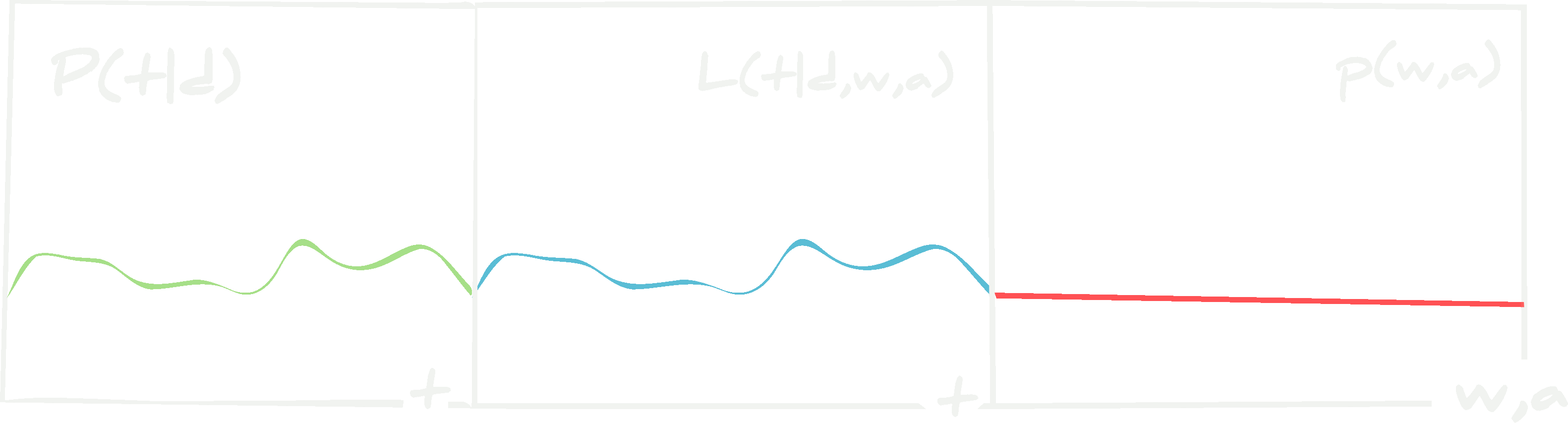The form of the posterior predictive density of the targets $${\bf t}$$ depends mostly on the probability of the weights and hyperparameters of the network. This means that the prior for the weights and hyperparameters must be chosen carefully or the posterior extremely well characterised via training data.

A Bayesian neural network is a network which provides the true posterior predictive density of targets $${\bf t}$$ given data $${\bf d}$$.

## Failure of traditionally trained neural networks

As described above, given a set of training pairs, $$\{ {\bf d}^\textrm{train}_i, {\bf t}^\textrm{train}_i\vert i\in[1, n_\textrm{train}]\}$$, and validation pairs, $$\{ {\bf d}^\textrm{val}_i, {\bf t}^\textrm{val}_i\vert i\in[1, n_\textrm{val}]\}$$, we can find the (local) maximum likelihood estimates of the weights, $$\boldsymbol{w}=\boldsymbol{w}^\textrm{local MLE}$$, and optimise the hyperparameters to $$\boldsymbol{\alpha}=\boldsymbol{\alpha}^*$$ which gives the best fit to both the training and validation data-target pair sets. Since we fix both the parameters and hyperparameters, those values are set in stone and we degenerate the posterior distribution to a Dirac $$\delta$$ function, neglecting any information brought by the training data, i.e.

\begin{align} \mathcal{P}(\boldsymbol{w},\boldsymbol{\alpha}|\{ {\bf d}^\textrm{train}_i, {\bf t}^\textrm{train}_i\vert i\in[1, n_\textrm{train}]\}) &\propto\mathcal{L}(\boldsymbol{w},\boldsymbol{\alpha}\vert \{ {\bf d}^\textrm{train}_i, {\bf t}^\textrm{train}_i\vert i\in[1, n_\textrm{train}]\})p(\boldsymbol{w},\boldsymbol{\alpha})\\ &\to\delta(\boldsymbol{w}-\boldsymbol{w}^\textrm{local MLE}, \boldsymbol{\alpha}-\boldsymbol{\alpha}^*) \end{align}

where $$p(\boldsymbol{w},\boldsymbol{\alpha})$$ is a prior distribution over the weights and hyperparameters. By making such a choice, we erase the entirety of the information about the distribution of data and work only with the best fit model, which may (or may not) be complete. As such, the predictive probability density of the targets $${\bf t}$$ given data $${\bf d}$$ is

$\mathcal{P}({\bf t}\vert {\bf d}) =\delta({\bf t}-\boldsymbol{\tau}({\bf d})),$

i.e., the probability of obtaining an estimate from the network is zero everywhere apart from at the value of the output of the network, $$\mathbb{NN}(\boldsymbol{w}^\textrm{local MLE}, \boldsymbol{\alpha}^*) : {\bf d}\to\boldsymbol{\tau}$$ - the function is completely deterministic. Effectively, this means that the probability of obtaining $${\bf t}$$ given the fixed network parameters and hyperparameters and some data $${\bf d}$$ is impossibly small.

Consider a third test set, $$\{ {\bf d}^\textrm{test}_i, {\bf t}^\textrm{test}_i\vert i\in[1, n_\textrm{test}]\}$$. One normally determines how well a neural network is trained using this unseen (blind) set. To test the network, all of the test data, $$\{ {\bf d}^\textrm{test}_i\vert i\in[1, n_\textrm{test}]\}$$, are passed through the network to get estimates $$\{\boldsymbol{\tau}^\textrm{test}_i\vert i\in[1, n_\textrm{test}]\}$$ which can be plotted against the known targets, $$\{ {\bf t}^\textrm{test}_i\vert i\in[1, n_\textrm{test}]\}$$ (see above figure).For any set of data, a trained neural network with fixed hyperparameters and network parameters at their maximum likelihood values, the probability of obtaining a target is a $$\delta$$ function. There is no knowledge of whether the output of the network will be equal to the target, and it is, in fact, improbably unlikely that they will be.

A network which produces $$\{\boldsymbol{\tau}^\textrm{test}_i\vert i\in[1, n_\textrm{test}]\}$$ which correlate very strongly with $$\{ {\bf t}^\textrm{test}_i\vert i\in[1, n_\textrm{test}]\}$$ is probably a network that is in a very good local maximum for both the weights and the hyperparameters. However, there is no assurance that the true $${\bf t}$$ should be obtained by the network, and due to the complexity of the likelihood $$\mathcal{L}({\bf t}\vert {\bf d}, \boldsymbol{w}, \boldsymbol{\alpha})$$, there is also no way of ensuring that $$\boldsymbol{\tau}$$ should be similar to $${\bf t}$$. Simply, for complex models, it is not possible to prove that the neural network is equivalent to the model, $$\mathbb{NN}(\boldsymbol{w},\boldsymbol{\alpha})\equiv\mathcal{M}$$, and so there is no trust that the network will provide $$\boldsymbol{\tau}={\bf t}$$. In fact, because $$\mathcal{P}({\bf t}\vert {\bf d})=\delta(\boldsymbol{\tau})$$, it is improbably unlikely to ever find $$\boldsymbol{\tau}={\bf t}$$. For extremely simple architectures it may be possible to prove that at the global maximum likelihood estimates of the weights that $$\mathbb{NN}(\boldsymbol{w},\boldsymbol{\alpha})\equiv\mathcal{M}$$, but unfortunately, such simple networks are much less likely to contain the exact representation of $$\mathcal{M}$$. Therefore, one can only prove $$\mathbb{NN}(\boldsymbol{w},\boldsymbol{\alpha})\equiv\mathcal{M}$$ in the limit of infinite data. This is because, in the limit of infinite training data and infinite validation data then we can assume (but not know) that a network could be found (via optimising the hyperparameters over the space of all possible architectures, activation functions, initial conditions of the weights, etc.) which has the capability to exactly reproduce the model $$\mathcal{M} : {\bf d}\to{\bf t}$$ by finding the true global maximum of the weights over the space of all possible weights in all possible architectures.

An interesting point to make, especially for regression to model parameters, is that one attempts to use the neural network to find a mapping from a many-to-one value space since the same $${\bf t}$$ could produce a very large number of different $${\bf d}$$, i.e. the forward model is stochastic. It is an extremely difficult procedure to undo stochastic processes, which is why the neural network will likely never achieve the target function.

## Variational inference using approximate weight priorsA neural network can be trained via variational inference where parameters of the network predict the parameters of a variational distribution from which the weights for the forward propagation are drawn.

All of the problems with the traditional picture arise due to degenerating the probability of the weights and hyperparameters $$\mathcal{P}(\boldsymbol{w}, \boldsymbol{\alpha})\to\delta(\boldsymbol{w}-\boldsymbol{w}^\textrm{local MLE}, \boldsymbol{\alpha}-\boldsymbol{\alpha}^*)$$. We can recover variational inference by assuming the posterior distribution of the weights becomes an approximate variational distribution, $$\mathcal{Q}(\boldsymbol{w}\vert \boldsymbol{v}, \boldsymbol{\alpha}, \{ {\bf d}^\textrm{train}_i, {\bf t}^\textrm{train}_i\vert i\in[1, n_\textrm{train}]\})$$, which approximates posterior of $$\boldsymbol{w}$$ given a secondary set of network parameters which define the shape of the variational distribution, $$\boldsymbol{v}$$, a set of hyperparameters, $$\boldsymbol{\alpha}$$, and a set of training data and target pairs, $$\{ {\bf d}^\textrm{train}_i, {\bf t}^\textrm{train}_i\vert i\in[1, n_\textrm{train}]\}$$. The posterior predictive density for the targets $${\bf t}$$ is then written

$\mathcal{P}({\bf t}\vert {\bf d})=\int d\boldsymbol{w}d\boldsymbol{v}d\boldsymbol{\alpha}~\mathcal{L}({\bf t}\vert {\bf d},\boldsymbol{w},\boldsymbol{\alpha})\mathcal{Q}(\boldsymbol{w}\vert \boldsymbol{v}, \boldsymbol{\alpha}, \{ {\bf d}^\textrm{train}_i, {\bf t}^\textrm{train}_i\vert i\in[1, n_\textrm{train}]\})p(\boldsymbol{v},\boldsymbol{\alpha}).$

In practice, the parameters controlling the shape of the variational distribution, $$\boldsymbol{v}$$ and the hyperparameters, $$\boldsymbol{\alpha}$$ are optimised iteratively using a training and validation set as with the traditional training framework and as such the posterior predictive density becomes

\begin{align} \mathcal{P}({\bf t}\vert {\bf d})&=\int d\boldsymbol{w}d\boldsymbol{v}d\boldsymbol{\alpha}~\mathcal{L}({\bf t}\vert {\bf d},\boldsymbol{w},\boldsymbol{\alpha})\mathcal{Q}(\boldsymbol{w}\vert \boldsymbol{v}, \boldsymbol{\alpha}, \{ {\bf d}^\textrm{train}_i, {\bf t}^\textrm{train}_i\vert i\in[1, n_\textrm{train}]\})\\ &\phantom{=hello}\times\delta(\boldsymbol{v}-\boldsymbol{v}^\textrm{local MLE}, \boldsymbol{\alpha}-\boldsymbol{\alpha}^*)\\ &=\int d\boldsymbol{w}~\mathcal{L}({\bf t}\vert {\bf d},\boldsymbol{w}, \boldsymbol{\alpha}^*)\mathcal{Q}(\boldsymbol{w}\vert \boldsymbol{v}^\textrm{local MLE}, \boldsymbol{\alpha}^*, \{ {\bf d}^\textrm{train}_i, {\bf t}^\textrm{train}_i\vert i\in[1, n_\textrm{train}]\}). \end{align}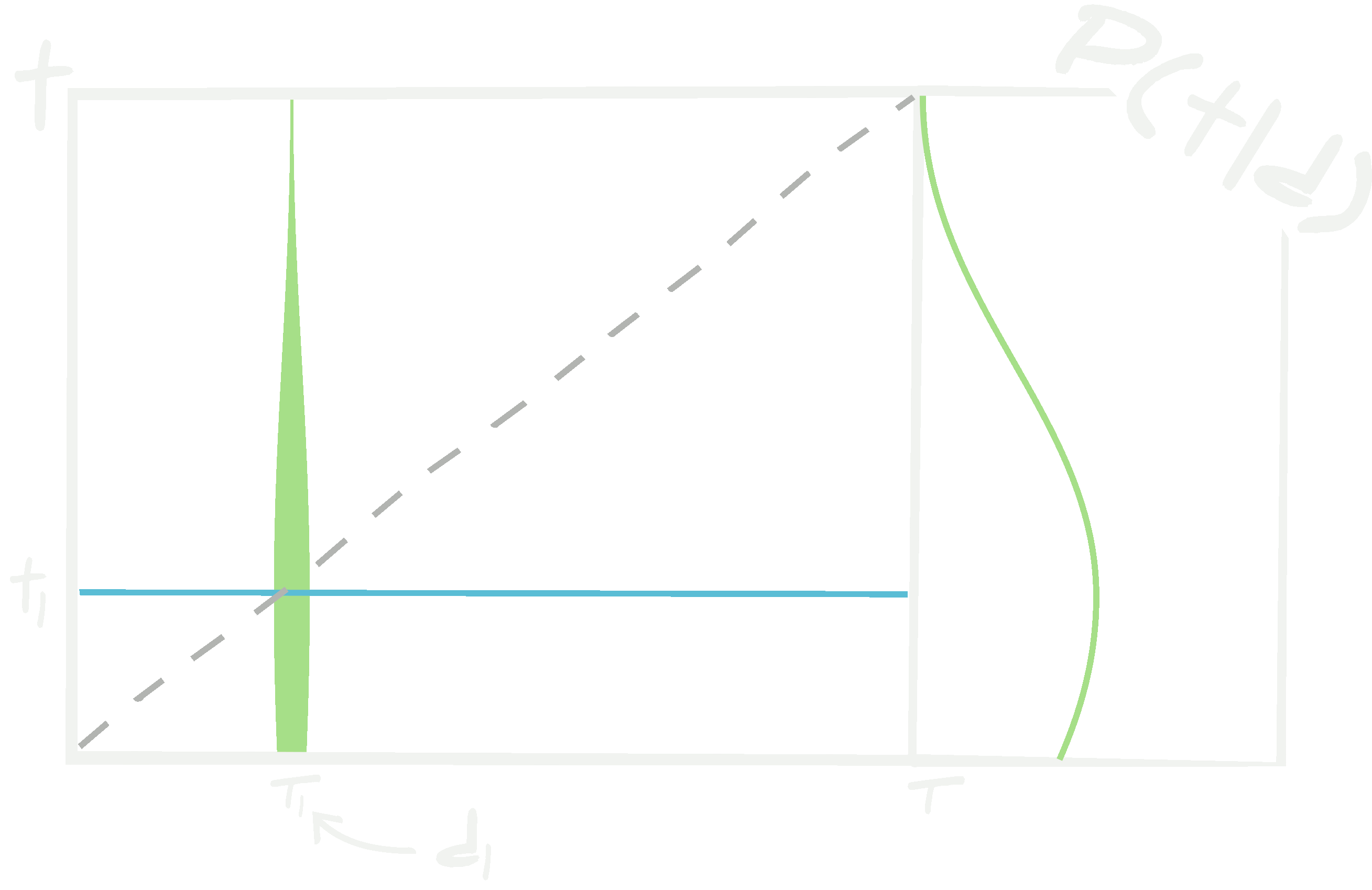When the posterior distribution for the weights and hyperparameters of a neural network are approximated using a variational distribution, the posterior predictive density for the targets given some data has a form dictated mostly by the shape of the variational distribution. This shape is not necessarily correct since only simple distributions are usually used for the variational distribution and the distribution of weights can be extremely complex.

In principle, if $$\mathcal{Q}(\boldsymbol{w}\vert \boldsymbol{v}^\textrm{local MLE}, \boldsymbol{\alpha}^*, \{ {\bf d}^\textrm{train}_i, {\bf t}^\textrm{train}_i\vert i\in[1, n_\textrm{train}]\})$$ well represents the true posterior of the weights and hyperparameters, $$\mathcal{P}(\boldsymbol{w}, \boldsymbol{\alpha})$$, then this can be a good approximation. However, this is very dependent on the distributions which $$\mathcal{Q}(\boldsymbol{w}\vert \boldsymbol{v}, \boldsymbol{\alpha})$$ can represent. $$\mathcal{Q}(\boldsymbol{w}\vert \boldsymbol{v}, \boldsymbol{\alpha})$$ is normally chosen to be Gaussian, or perhaps a mixture of Gaussians. As discussed already, the likelihood of obtaining any set of weights, $$\boldsymbol{w}$$, is actually extremely bumpy and degenerate and, as such, $$\mathcal{Q}(\boldsymbol{w}\vert \boldsymbol{v}, \boldsymbol{\alpha})$$ must be chosen to be able to properly represent this. If $$\mathcal{Q}(\boldsymbol{w}\vert \boldsymbol{v}, \boldsymbol{\alpha})$$ is poorly proposed then the posterior predictive density of the targets, $$\mathcal{P}({\bf t}\vert {\bf d})$$, will be incorrect.The variational distribution often does not have enough complexity to fully model the intricate nature of the true posterior distribution of weights and hyperparameters. This can lead variational inference te be misleading.

## Bayesian neural networksA Bayesian neural network is similar a traditional one apart from the distribution of the weights (and hyperparameters) of the network are characterised by the posterior for the weights and hyperparameters given a set of training data.

An effective Bayesian neural network can be be built if we use the true posterior of $$\boldsymbol{w}$$ and $$\boldsymbol{\alpha}$$ given some training data, rather than degenerating it to a Dirac $$\delta$$, and instead keeping

$\mathcal{P}(\boldsymbol{w},\boldsymbol{\alpha}\vert \{ {\bf d}^\textrm{train}_i, {\bf t}^\textrm{train}_i\vert i\in[1, n_\textrm{train}]\})\propto\prod_i^{n_\textrm{train} }\mathcal{L}({\bf t}^\textrm{train}_i\vert {\bf d}^\textrm{train}_i,\boldsymbol{w},\boldsymbol{\alpha})p(\boldsymbol{w},\boldsymbol{\alpha}).$

With this, the predictive probability density of $${\bf t}$$ given $${\bf d}$$ becomes

\begin{align} \mathcal{P}({\bf t}\vert {\bf d}) =&~\int d\boldsymbol{w}d\boldsymbol{\alpha}~\mathcal{L}({\bf t}\vert {\bf d}, \boldsymbol{w}, \boldsymbol{\alpha})\mathcal{P}(\boldsymbol{w},\boldsymbol{\alpha}\vert \{ {\bf d}^\textrm{train}_i, {\bf t}^\textrm{train}_i\vert i\in[1, n_\textrm{train}]\})\\ \propto&~\int d\boldsymbol{w}d\boldsymbol{\alpha}~\mathcal{L}({\bf t}\vert {\bf d}, \boldsymbol{w}, \boldsymbol{\alpha})\prod_i^{n_\textrm{train} }\mathcal{L}({\bf t}^\textrm{train}_i\vert {\bf d}^\textrm{train}_i,\boldsymbol{w},\boldsymbol{\alpha})p(\boldsymbol{w},\boldsymbol{\alpha}). \end{align}

Obviously the Bayesian neural network comes at a much higher computational cost than just finding the maximum likelihood estimate for the weights, but it does provide a more reasoned posterior predictive probability density, $$\mathcal{P}({\bf t}\vert {\bf d})$$. Notice that the prior, $$p(\boldsymbol{w},\boldsymbol{\alpha})$$, still enters and so we need to make an informed decision on our belief for what the values of $$\boldsymbol{w}$$ and $$\boldsymbol{\alpha}$$ should be. However, for enough training data-target pairs (and enough time to sample through whatever chosen prior, $$p(\boldsymbol{w},\boldsymbol{\alpha})$$) the posterior $$\mathcal{P}(\boldsymbol{w},\boldsymbol{\alpha}\vert \{ {\bf d}^\textrm{train}_i, {\bf t}^\textrm{train}_i\vert i\in[1, n_\textrm{train}]\})$$ becomes informative enough to obtain useful posterior predictions for the targets.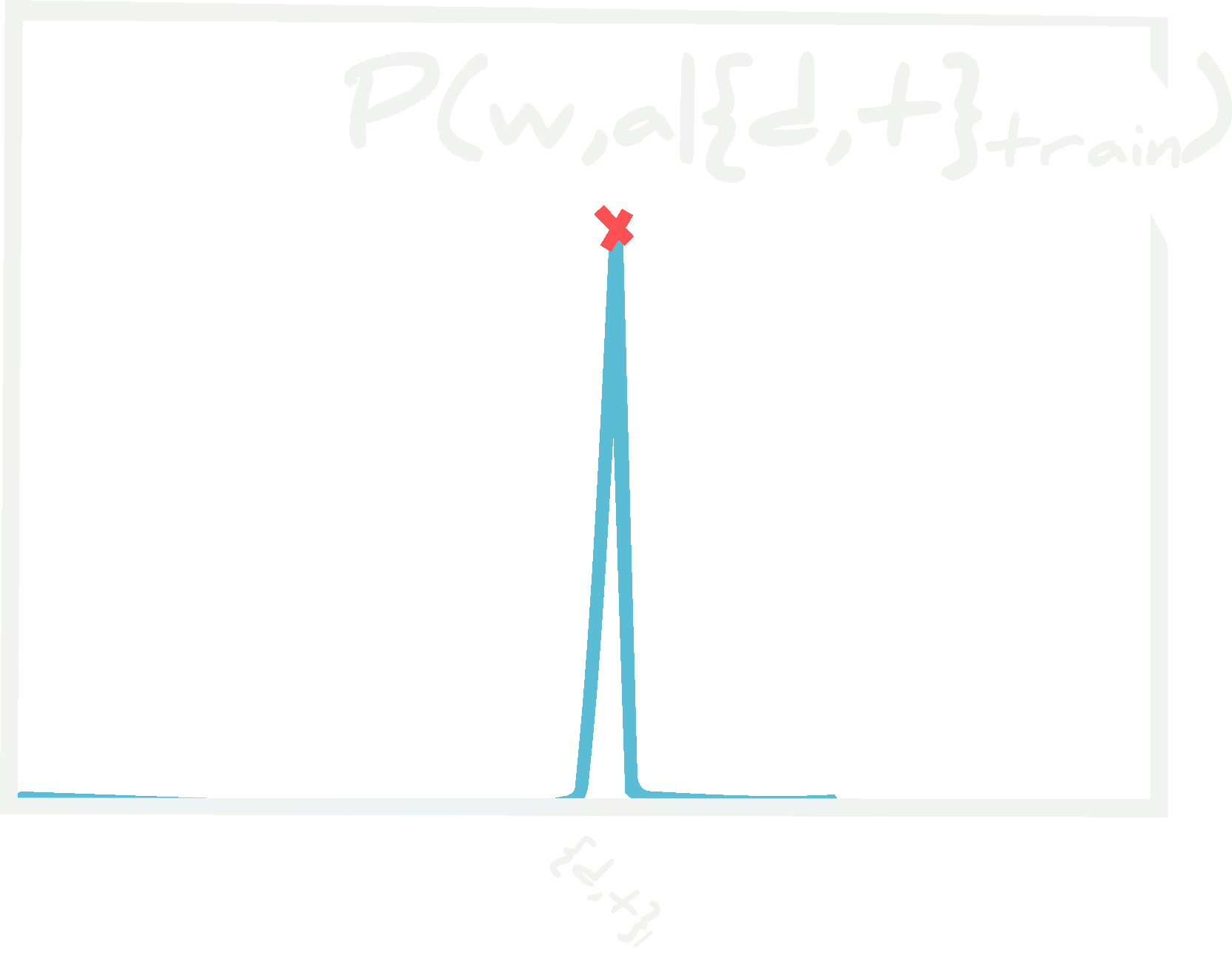For small numbers of data points, the likelihood is poorly characterised and so can lead to biasing in the posterior predictive density. It is therefore important to have enough data to properly know the likelihood - it is not easy to determine how much this is.

In effect, to make use of Bayesian neural networks, one has to resort to sampling techniques, such as Markov chain Monte Carlo, to describe $$\mathcal{P}({\bf t}\vert {\bf d})$$. Because of the (normally extremely large) dimension of the number of weights, techniques such as Metropolis-Hastings cannnot be considered. We proposed using a second-order geometrical adaptation of Hamiltonian Monte Carlo (QN-HMC) in Charnock et al. 2019 (read more). By using such a sampling technique, one could generate samples for the posterior predictive density, $$\mathcal{P}({\bf t}\vert {\bf d})$$, whose distribution describes what was the probability of getting a target $${\bf t}$$ from data $${\bf d}$$ marginalised over all network parameters $$\boldsymbol{w}$$ given a hyperparameter, $$\boldsymbol{\alpha}=\boldsymbol{\alpha}^*$$1. It is difficult to sample $$\boldsymbol{\alpha}$$ when using the QN-HMC since gradients of the likelihood need to be computed and the likelihood in the $$\boldsymbol{\alpha}$$ direction is often discrete. How to properly sample from $$\boldsymbol{\alpha}$$ is still up for debate.

So now lets say we have enough computational power to build a true Bayesian neural network. Are we guaranteed to obtain a correct posterior predictive density?

# Source of the problem

## Training on data

Notice how all of the techniques mentioned above are dependent on a set of training data and target pairs, $$\{ {\bf d}^\textrm{train}_i, {\bf t}^\textrm{train}_i\vert i\in[1, n_\textrm{train}]\}$$ (and possibly validation data and targets, $$\{ {\bf d}^\textrm{val}_i, {\bf t}^\textrm{val}_i\vert i\in[1, n_\textrm{val}]\}$$). It is in the posterior (or variational distribution) for the weights that the training data arises

$\mathcal{P}({\bf t}\vert {\bf d}) = \int d\boldsymbol{w}d\boldsymbol{\alpha}~\mathcal{L}({\bf t}\vert {\bf d}, \boldsymbol{w}, \boldsymbol{\alpha})\mathcal{P}(\boldsymbol{w},\boldsymbol{\alpha}\vert \{ {\bf d}^\textrm{train}_i, {\bf t}^\textrm{train}_i\vert i\in[1, n_\textrm{train}]\})$

and, as already explained, the last term in the integral contains the informative part about the posterior predictive density. As such, any biasing due to $$\{ {\bf d}^\textrm{train}_i, {\bf t}^\textrm{train}_i\vert i\in[1, n_\textrm{train}]\}$$ greatly affects $$\mathcal{P}({\bf t}\vert {\bf d})$$.

When depending on a training set, $$\mathcal{P}({\bf t}\vert {\bf d})$$ is always unknowably biased until the limit of infinite data is reached. So, no method mentioned so far provides us with the correct probability of obtaining the target!

For networks, such as emulators (or generative networks as they are commonly called), where the probability distribution of generating targets, $$\mathcal{\bf P}({\bf t}\vert {\bf z})$$, with generated data $${\bf t}$$ and a latent distribution $${\bf z}$$, should approximate the distribution of true data $$\mathcal{P}({\bf d})$$, then the above argument means that we cannot find $$\mathcal{P}({\bf d})$$ by training a neural network without infinite training data2.

## Incorrect models

One interesting use for neural networks is the predicting of physical model parameters, $$\boldsymbol{\theta}$$, for a model $$\mathcal{M} : \boldsymbol{\theta}\to{\bf d}$$. In this case, even for infinite data, we cannot obtain true posterior distributions for the parameters. Take a network which maps $$\mathbb{NN}(\boldsymbol{w},\boldsymbol{\alpha}) : {\bf d}\to\hat{\boldsymbol{\theta}}$$, where $$\hat{\boldsymbol{\theta}}$$ are estimates of the model parameters, $$\boldsymbol{\theta}$$, which generate the data. Even if there is infinite training data, $$\{ {\bf d}^\textrm{train}_i, \boldsymbol{\theta}^\textrm{train}_i\vert i\in[1,\infty]\}$$, if the original model is incorrect, then the neural network will be conditioned on the wrong map from data, $${\bf d}$$, to parameters, $$\boldsymbol{\theta}$$, and so any observed data, $${\bf d}^\textrm{obs}$$, passed through the network will be passed through the incorrect approximation of the model and provide a poor estimate of the incorrect model parameter values. This means that true posteriors on the model parameters can only be obtained with the exact model which generates the observed data and an infinite amount of training data from that model, to be able to correctly provide parameter estimates.

This is not realistic!

# Solutions

We have so far built a description of how to obtain the probability to obtain targets, $${\bf t}$$, from data, $${\bf d}$$, passed through a neural network… and unfortunately, we have learned that it is not possible to obtain.

There is still one problem where we can use neural networks safely despite all of the above. This is to do model parameter inference.

So far we have only considered a neural network as an approximation to a model $$\mathcal{M} : {\bf d}\to{\bf t}$$. Now lets say we have a physical model, $$\mathcal{Z}(\boldsymbol{\iota}) : \boldsymbol{\theta}\to{\bf d}$$, which generates the data, $${\bf d}$$ from a set of model parameters, $$\boldsymbol{\theta}$$, dependent on a set of initial conditions $$\boldsymbol{\iota}$$, we can safely use a neural network, $$\mathbb{N}(\boldsymbol{w},\boldsymbol{\alpha}) : {\bf d}\to\boldsymbol{\tau}$$, to infer the model parameters of some observed data, $${\bf d}^\textrm{obs}$$. Note that we cannot use a network to predict model parameters directly $$(\mathbb{NN} : {\bf d}\to\boldsymbol{\theta})$$ due to all of the arguments above. Instead we need to set up a statistical inference framework which encompasses the neural network.

Charnock et al. 2019 and Charnock, Lavaux and Wandelt 2018 show two different methods to perform physical model parameter inference using neural networks, in a well justified way.

## Writing down the likelihood

I should mention an extremely rare case where the model $$\mathcal{M} : {\bf d}\to{\bf t}$$, is simple enough to be parameterised by an extremely simple network with very few parameters, which are non-degenerate and well behaved and for which the hyperparameters, $$\boldsymbol{\alpha}$$, can be well designed to avoid needing to sample over this space.

For this case, the likelihood could be written, and therefore, fully established and sampled from, and biases from training data-target pairs could be totaly avoided.

It is pretty unlikely that such a network could be found without considering physical principles.

## Model extension

In Charnock et al. 2019, the connection between the observed data and the output of the physical model is not known, i.e. the data from a model given initial conditions, $$\boldsymbol{\iota}$$, is $$\mathcal{Z}(\boldsymbol{\iota}) : \boldsymbol{\theta}\to{\bf d}$$. This $${\bf d}$$ does not look like $${\bf d}^\textrm{obs}$$ although we know that want the posterior distribution of $$\mathcal{P}(\boldsymbol{\theta}\vert {\bf d}^\textrm{obs})$$. In Charnock et al. 2019, we know we can observe the universe and model the underlying dark matter of the universe, but the complex astrophysics which maps the dark matter of the universe to the observable tracers is unknown. We do, however, know some physical properties of this mapping. In this case, we build a neural network with the physically motivated symmetries to take the output of the physical model to the distribution which is as close to the observed data as possible (read more). In the language used previously, thanks to the problems we deal with in cosmology and astrophysics we can actually choose the hyperparameters of a neural network, $$\boldsymbol{\alpha}$$, in a reasoned manner. These physically motivated neural networks therefore massively reduce the volume of the $$\boldsymbol{\alpha}$$ domain. With a careful choice of $$\boldsymbol{\alpha}$$ we can also build a network whose priors on the network paremeters, $$\boldsymbol{w}$$, can be (at least reasonably) well informed.

We can write the parameter inference as

$\mathcal{P}(\boldsymbol{\theta}\vert {\bf d}^\textrm{obs}) \propto \int d\boldsymbol{\iota}d\boldsymbol{w}d\boldsymbol{\alpha}~\mathcal{L}({\bf d}^\textrm{obs}\vert \boldsymbol{\iota},\boldsymbol{w},\boldsymbol{\alpha})\mathcal{P}(\boldsymbol{\iota}\vert \boldsymbol{\theta})p(\boldsymbol{w},\boldsymbol{\alpha})$

That is, the posterior distribution for the model parameters given some observed data is proportional to the marginal distribution of how likely the observed data is given the initial conditions of the model, $$\boldsymbol{\iota}$$, which depend on the model parameters, $$\boldsymbol{\theta}$$, which generate the initial conditions and evolve the model forward to the input of the neural network with network parameters and hyperparameters $$\boldsymbol{w}$$ and $$\boldsymbol{\alpha}$$.

In this presented case, there is no training data for the network, instead the data needed to obtain the posterior is part of the statistical framework. Therefore, the network provides non-agnostic posterior parameter inference because we do not learn the posterior distribution, $$\mathcal{P}(\boldsymbol{w},\boldsymbol{\alpha})$$ using training data. In essence, this defines the procedure to perform zero-shot training.

It should be noted that this procedure is difficult. It necessitates a sampling scheme for the neural network and the physical model. In Charnock et al. 2019, we use an advanced Hamiltonian Monte Carlo sampling technique on a model where we have calculated the adjoint gradient and the neural network whose architecture is well informed but fixed.

## Likelihood-free inference

The model extension method works well, but still depends on knowing the form of the likelihood of the observed data. In practice, this could be extremely difficult. It also depends on a choice of hyperparameters (or at least a well defined prior based on physical principles). In Charnock, Lavaux and Wandelt 2018, we showed another model extension method which allows use to obtain optimal model parameter inference using neural networks by (semi)-classically training a neural network, $$\mathbb{NN}(\boldsymbol{w},\boldsymbol{\alpha}) : {\bf d}\to{\bf t}$$, where the target distribution is the set of Gaussianly distributed summaries which maximise the Fisher information matrix. Although the network in this work is, in some way, optimal - the main point of this paper is that parameter inference can be done using likelihood-free inference by extending the physical model $$\mathcal{M} : \boldsymbol{\theta}\to{\bf d}$$ to $$\mathcal{N} :\boldsymbol{\theta}\to{\bf t}$$ where $${\bf t}$$ is any set of summaries.

Likelihood-free inference is a framework where, via generating data using the physical model, $$\mathcal{M} : \boldsymbol{\theta}\to{\bf d}$$, the joint probablity of data and parameters, $$\mathcal{P}({\bf d},\boldsymbol{\theta})$$, can be characterised. Once this space is well defined, a slice through the distribution at any $${\bf d}^\textrm{obs}$$ gives the posterior distribution $$\mathcal{P}(\boldsymbol{\theta}\vert {\bf d}^\textrm{obs})$$ - likewise the slice through the joint distribution at any parameter $$\boldsymbol{\theta}^*$$ gives the likelihood distribution $$\mathcal{L}({\bf d}\vert \boldsymbol{\theta^*})$$. This works for any system where we can model the data!

The neural networks become essential as functions which perform data compression (although, it should be noted that any summary of the data will work). Since, in general, the dimensionality of the data is much larger than the number of model parameters, a neural network can be trained to compress the data in some way, $$\mathbb{NN}(\boldsymbol{w}^*,\boldsymbol{\alpha}^*) : {\bf d}\to{\bf t}$$. We can train this in any way to give us some absolute summaries, $${\bf t}$$, where we, essentially, do not care what the summaries are. Note that $$\boldsymbol{w}$$ and $$\boldsymbol{\alpha}$$ do not need to be maximum likelihood estimates. By pushing all the generated data from the physical model through this fixed network we can characterise the probability distribution of parameters and compressed summaries, $$\mathcal{P}({\bf t},\boldsymbol{\theta})$$, which we can slice at any $$\boldsymbol{\theta}^*$$ to give the likelihood of obtaining any summaries, $$\mathcal{L}({\bf t}\vert \boldsymbol{\theta}^*)$$, or (more interestingly) slice at any observed data pushed through the network, $$\mathbb{NN}(\boldsymbol{w}^*, \boldsymbol{\alpha}^*) : {\bf d}^\textrm{obs}\to{\bf t}^\textrm{obs}$$, to get the posterior,

$\mathcal{P}(\boldsymbol{\theta}\vert {\bf t}^\textrm{obs})=\mathcal{P}(\boldsymbol{\theta}\vert {\bf d}^\textrm{obs},\boldsymbol{w}^*,\boldsymbol{\alpha}^*).$

This posterior, whilst conditional on the network parameters and hyperparameters, is unbiased in the sense that when the neural network, $$\mathbb{NN}(\boldsymbol{w}^*,\boldsymbol{\alpha}^*) : {\bf d}\to{\bf t}$$ is not optimal, the posterior can only become inflated (and not incorrectly biased).

The information maximising neural network, presented in Charnock, Lavaux and Wandelt 2018, provides the optimal summaries3 for the likelihood-free inference - but any neural network can be used in this inference framework. In particular, any neural network which looks like it provides good estimates of the targets for a model $$\mathcal{M} : {\bf d}\to{\bf t}$$ (as discussed throughout), will likely have extremely informative summaries, even if their outputs are improbably unlikely to be equal to the true target values (see traditionally training neural networks)!

# Conclusions

Presented here is a thorough statistical diagnostic of neural networks. I have shown that, by design, neural networks cannot provide realistic posterior predictive densities for arbitrary targets. This essentially makes all neural networks unusable in science.

However, I have presented how my previous works can undermine this previous statment for model parameter inference. Since either a statistical interpretation or a fully trained neural network can be appended to a physical model, we can build a statistical framework around both the model and the neural network to allow us to do rigorous, scientific analysis of model parameters, which is one of the essential tasks in science today.

Tom Charnock, Guilhem Lavaux, Benjamin D. Wandelt, Supranta Sarma Boruah, Jens Jasche, Michael J. Hudson, 2019, submitted to MNRAS, arXiv:1909.06379

Tom Charnock, Guilhem Lavaux, Benjamin D. Wandelt, 2018, Physical Review D 97, 083004 (2018), arxiv:1802.03537

1. It should be noted that the work in Charnock et al. 2019 was tackling a larger problem and asking a different question than the one stated here for Bayesian neural networks. Bayesian neural networks are a subset of the techniques from that paper, although closely linked.

2. We can hope that the generated target distribution gets close to the true data distribution and decide we are not bothered about statistics anymore. Maybe a dangerous situation for science‽

3. Optimal in the sense that the Fisher information is maximised. This has some assumptions such as the unimodality (but not necessarily Gaussianity) of the posterior, and the fact that the neural network being maximised is capable of finding a function which Gaussianises the data.

Authored by T. Charnock
Post identifier: /method/machine%20learning/nn